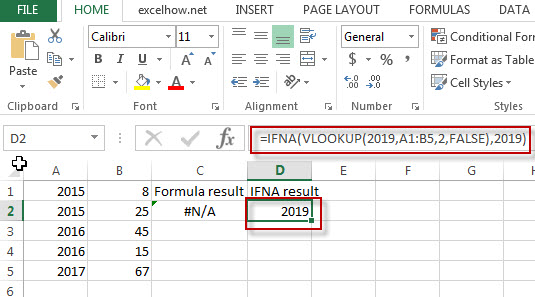# Excel IFNA Function

This post will guide you how to use Excel IFNA function with syntax and examples in Microsoft excel.

### Description

The Excel IFNA function returns an alternate value you specify if a formula results in an #N/A error, or returns the result of the formula.

The IFNA function is a build-in function in Microsoft Excel and it is categorized as a Logical Function.

The IFNA function is available in Excel 2016, Excel 2013, Excel 2010, Excel 2007, Excel 2003, Excel XP, Excel 2000, Excel 2011 for Mac.

### Syntax

The syntax of the IFNA function is as below:

= IFNA (value, value_if_error)

Where the IFNA function arguments are:
Value -This is a required argument. The value or formula that you want to test
Value_if_error – This is a required argument.  The value that is returned if the formula evaluates to a #N/A! error.

### Example

The below examples will show you how to use Excel IFNA Function to return an alternate value if a formula evaluates to a #N/A! error.

#1 =IFNA(VLOOKUP(2019,A1:B5,2,FALSE),2019)Note: the formula “VLOOKUP(2019,A1:B5,2,FALSE),” will return an #N/A! Error, if you use “IFNA” function, the above excel formula will return an alternate value “2019”.